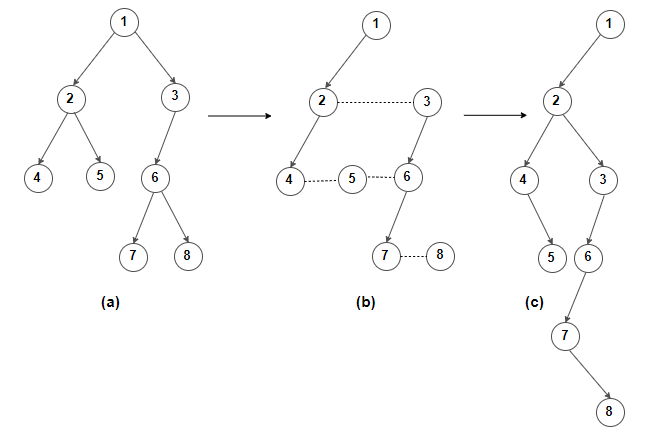# Convert binary tree to Left-child right-sibling binary tree

Given a normal binary tree, convert it to Left-child right-sibling (LC-RS) binary tree.

Each node in LC-RS binary tree has two pointers: one to the node’s left child, and one to its next sibling in original binary tree. So starting with the root, each node’s leftmost child in the original tree is made its left child in the LC-RS binary tree, and its nearest sibling to the right in the original tree is made its right child in the binary tree. For example, we have a binary tree below:(a) Processing binary tree to LC-RS binary tree, every node is linked and aligned with the left
child, and the next nearest is a sibling.

(b) We can re-write binary tree shown by putting the left child node to one level below its parents
and by putting the sibling next to the left child at the same level.

(c) We can transform this tree to a binary tree by turning each sibling 45° clockwise.

The idea is to traverse the tree in postorder fashion and for every node

• If its left child is empty, then make its right child as left’s and set right to null.
•

• If left child already exists, then make right child of its left child to point to its right child and set right child to null.

C++ implementation –

The time complexity of above solution is O(n) and auxiliary space used by the program is O(1).(1 votes, average: 5.00 out of 5)Loading...